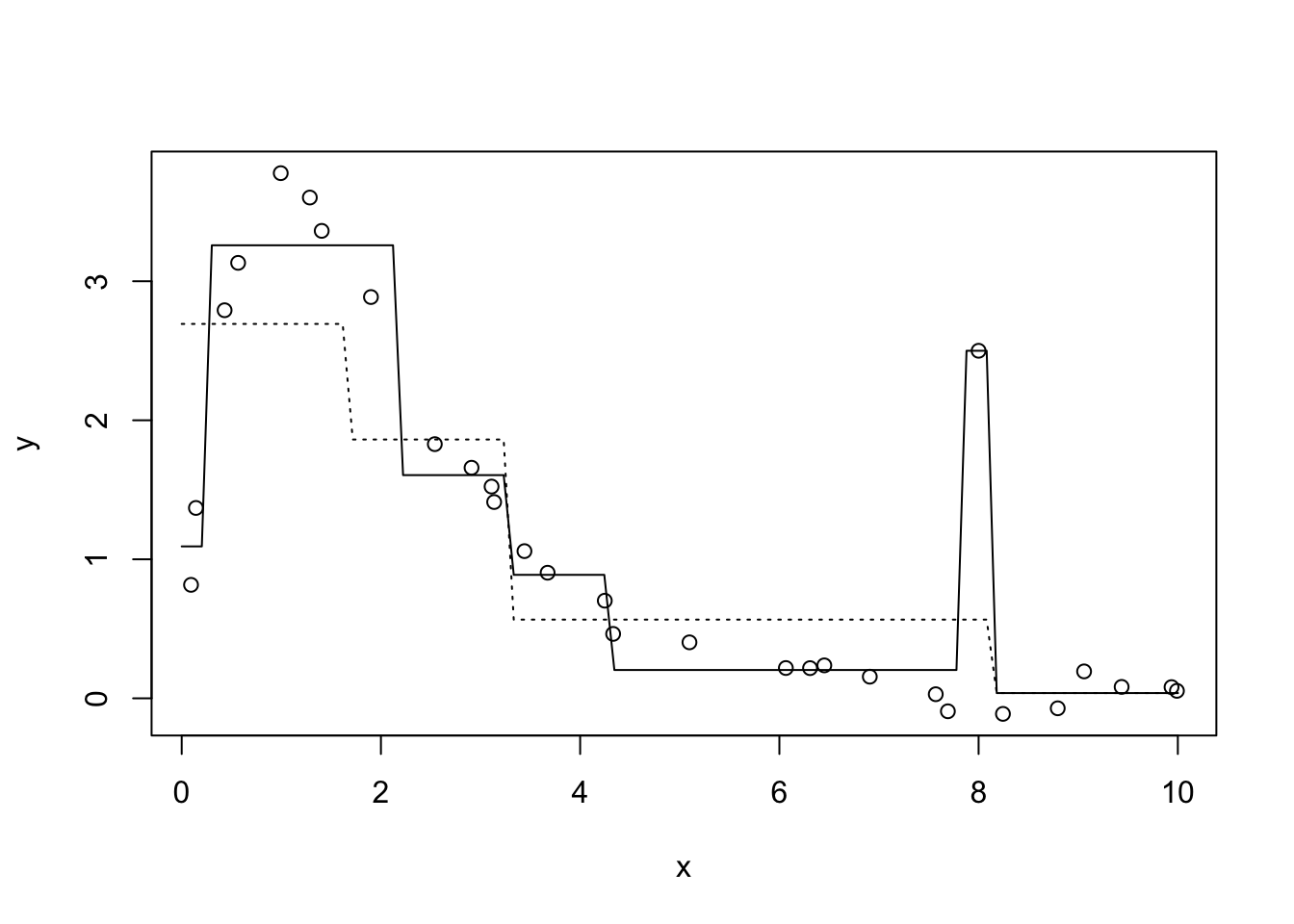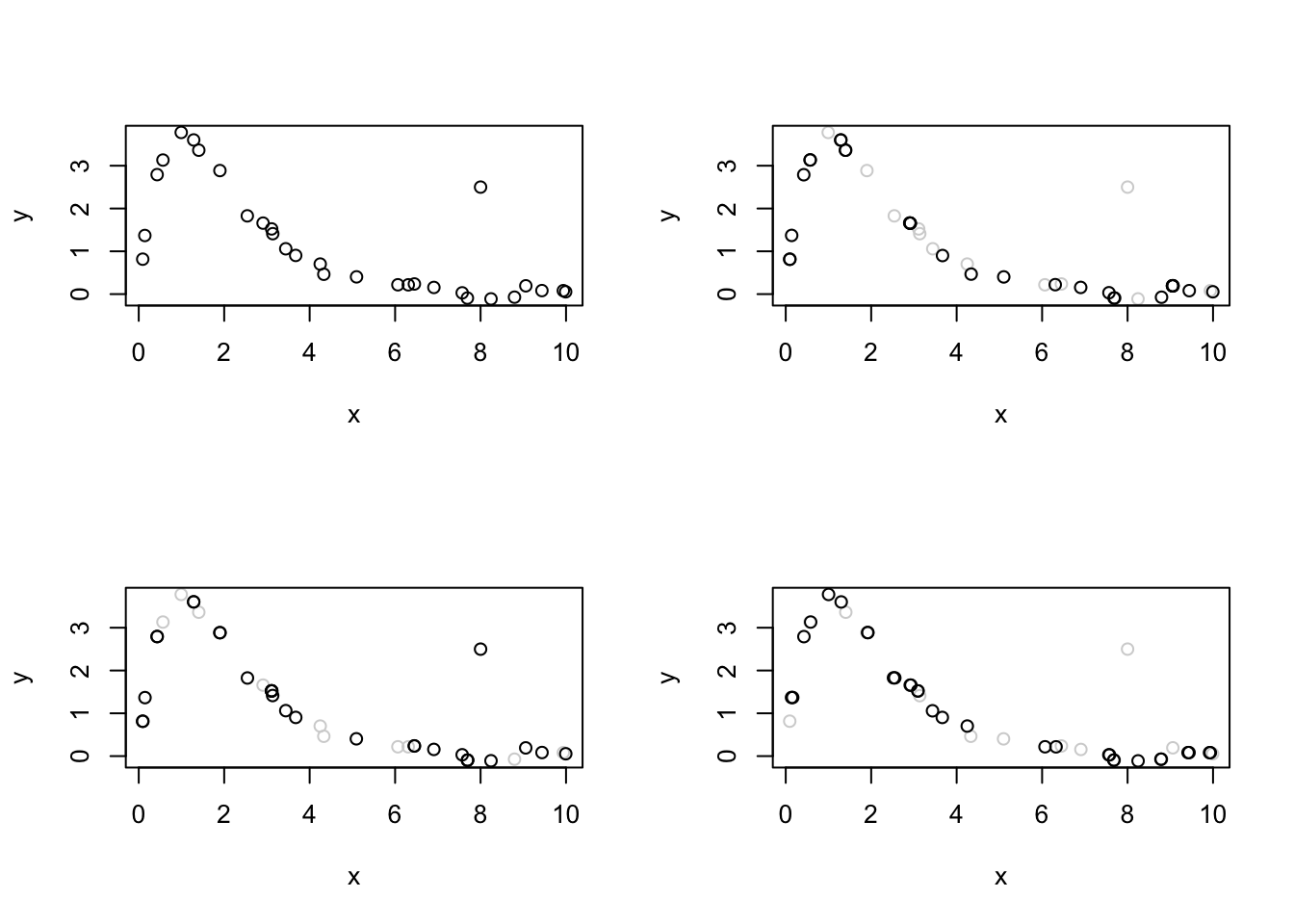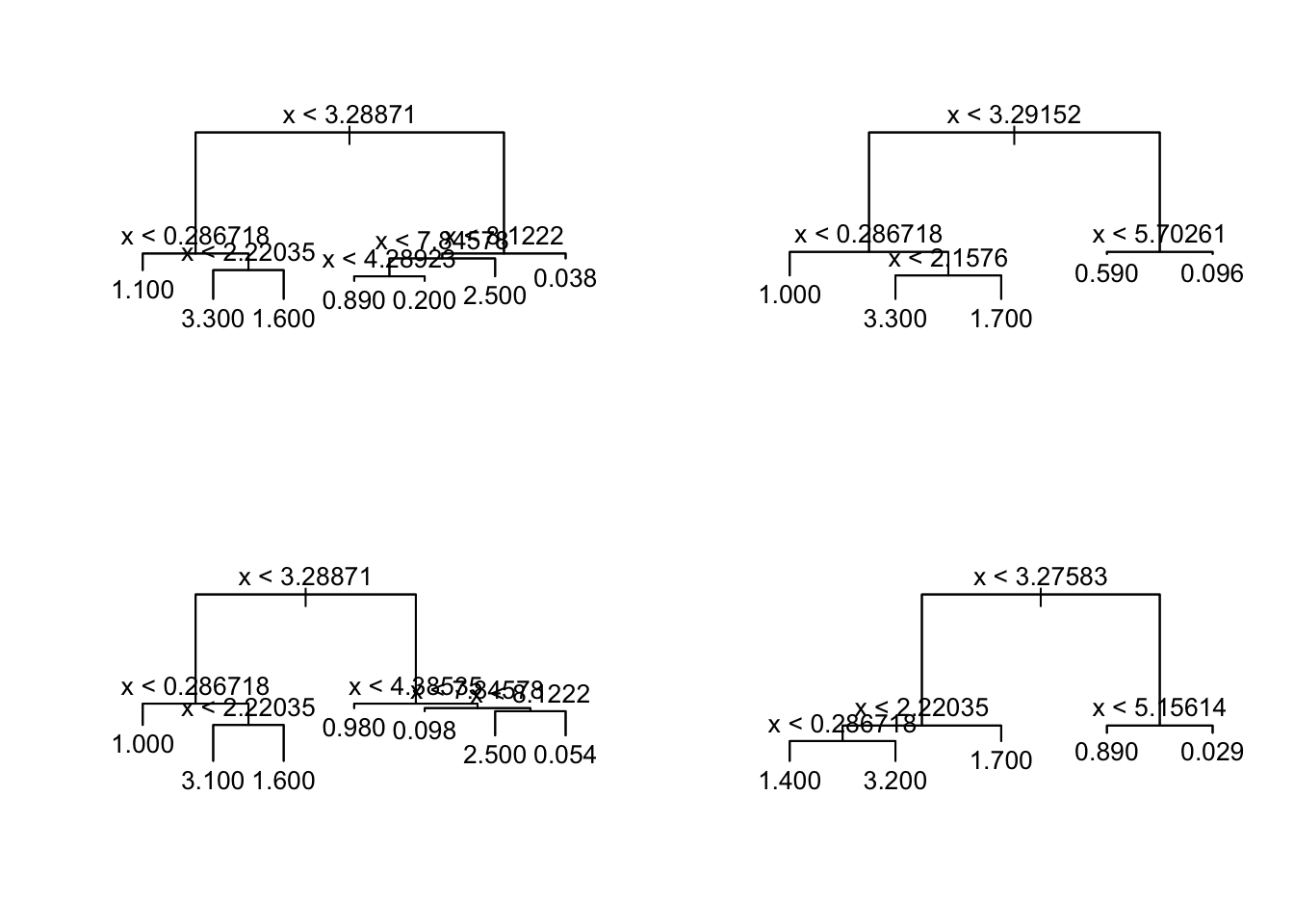$\newcommand{\Expect}{\mathbb{E}\left[ #1 \right]} \newcommand{\Var}{\mathrm{Var}\left[ #1 \right]} \newcommand{\Cov}{\mathrm{Cov}\left[ #1 \right]}$

# Trees

## Recap on the CART procedure for growing trees

• If there are less than $$n_{min}$$ cases left, stop
• Consider all possible binary splits based on one feature alone
• For classification outcomes, consider the reduction in $$H[Y|X]$$
• For regression, consider the reduction in $$\Var{Y|X}$$
• Does the best split improve the prediction by at least $$\delta$$?
• If no, stop
• If yes, recursively apply the procedure to cases on either side of the split
• Prune the tree by cross-validation

## Virtues and limits of trees

• Trees can approximate any function you like, to any degree of approximation you like
• Proof: It’s what you do to plot a curve on a screen with pixels
• Fully nonparametric, perfectly happy to handle interactions
• In fact, by default every variable gets to “interact” with every other
• Really good approximation may nonetheless need a really large number of leaves
• More leaves means less bias but also more variance
• True even if the splits are all treated as fixed
• Even more true if the splits are found by adaptively growing the treeSome simulated regression data (dots), plus a regression tree fit to the data by growing a big tree without pruning (solid line), and another regression tree fit with the default control settings (dotted line). Notice how the more stable, dotted-line tree misses the outlier (if it is an outlier and not a genuine feature of the data-generating process), but also misses some of the apparent structure of the regression curve (if it is structure and not just seeing patterns in noise).

• Getting really good performance may require a really big tree (with low bias), but those have very high variance
• How can we grow big trees with low variance?

# Forests

• “Forest” methods combine the predictions from multiple trees
• Forests are a special case of ensemble methods, which combine multiple models to improve on what any one of them could do
• The simplest sort of combination is averaging
• For classification, whenever I write “averaging”, read “voting”
• (Or, if you like, average the conditional probabilities and then threshold the average probability)

# Three leading forms of ensemble methods

1. “Bagging”: randomly perturb the data, grow a tree to the new data, average
2. “Random forests”: combine bagging with random feature selection
3. “Boosting”: sequentially fit models to the errors of earlier models

## Bagging, or “bootstrap averaging” (Breiman 1996)

The bagging procedure is simplicity itself:

• Start with a data set $$D = (X_1, Y_1), \ldots (X_n, Y_n)$$
• Fix the number of trees $$m$$ we want in the forest
• For $$k \in 1:m$$
• Generate $$\tilde{D}_k$$ by resampling the $$(X_i, Y_i)$$ $$n$$ times, with replacement
• That is, $$\tilde{D}_k$$ is a resampling (“nonparametric”) bootstrap simulation of the data-generating process
• With high probability, some data points are repeated in $$\tilde{D}_k$$, and some do not appear at all
• Grow a tree $$\tau_k$$ from $$\tilde{D}_k$$
• Typically without pruning
• Make predictions by averaging the $$\tau_k$$

### A little demoOriginal data for the running example (top left) and three bootstrap resamplings; in each resampling, the full data set is shown in light grey (for comparisons), and the coordinates are slightly “jittered”, so that a repeatedly-sampled point appears as multiple points very close to each other.Tree fit to the full data (top left), plus the three trees fit to the three bootstrap resamplings from the previous figure.